# Causal Graphical Model

## Directed Acyclic Graph (DAG)

Graph is a visual notation of relationship among a set of nodes, or vertices, and a set of edges which connects between nodes. The expression “Directed” means that each nodes have direction. And if there is a path from a node, goes back to the starting node throught directed edges, the path is called Cyclic. Without this, it is called Directed Acyclic Graph(DAG for short)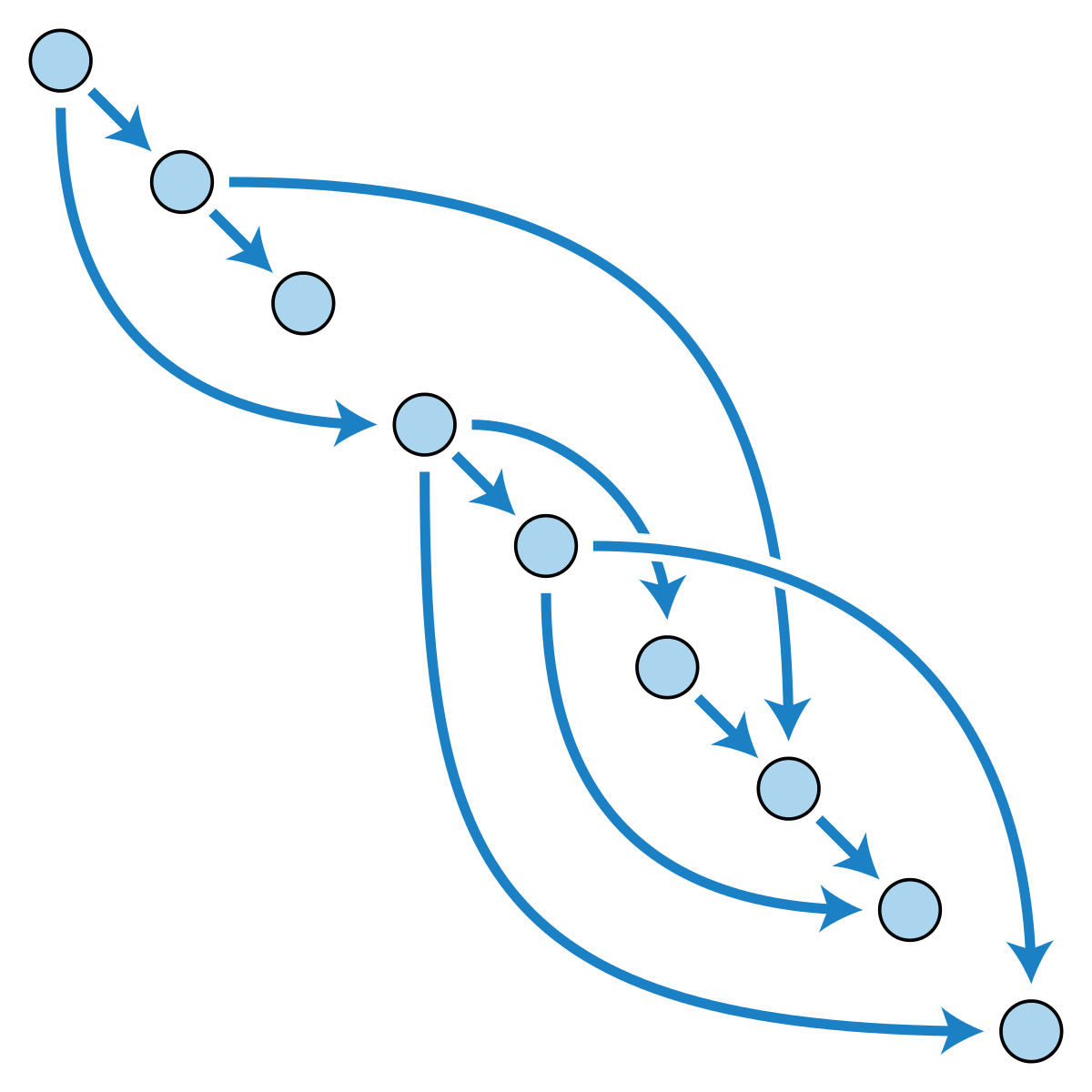Shown in figure, there is no path to return to the starting node throught directed edges, so this is DAG. Usually, we express them with hierarchical relationship, and the node placed in higher level is called ancestor, and node in lower level is called descendant.

## Conditional Dependency

Suppose we have random variable $X$ and $Y$. Its conditional probability of $X$ on $Y$ is defined as a joint probability of $X$ and $Y$ over probability of $Y$.

$P(X \vert Y) = \frac{P(X, Y)}{P(Y)}$

Here, we can assume $X$ and $Y$ are independent if these conditions are satisfied,

• $P(X \vert Y) = P(X)$
• $P(Y \vert X) = P(Y)$
• $P(X, Y) = P(X) \cdot P(Y)$

(actually those conditions are derived from the definition of conditional probability)

And it can be represented with following mathematical symbol

$X \perp Y$

Let’s look at three random variables $X, Y, Z$. In this case, $X$ and $Y$ are indepedent conditioned on $Z$ if this condition is safisfied,

$P(X, Y \vert Z) = P(X \vert Z) \cdot P(Y \vert Z)$

## Bayesian Networks

Why should we review the concept of DAG and conditional probabilities? That’s because the bayesian network is implemented with DAG and have specific properties related with conditional probabilities.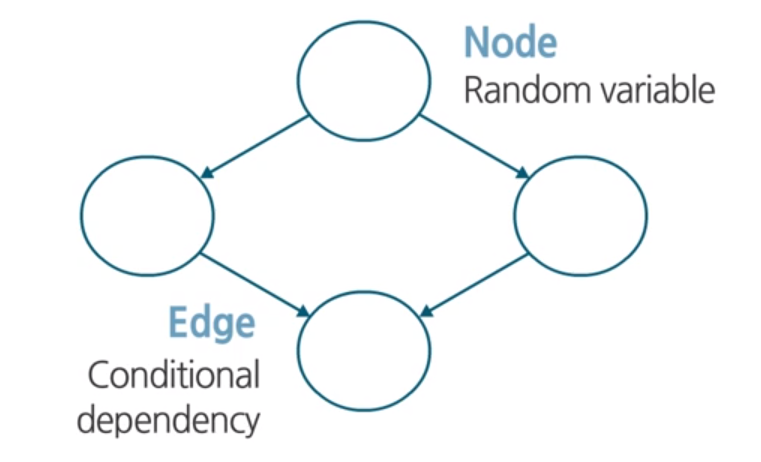Bayesian Networks is structured, graphical representation of probabilistic relationships between several random variables. Here, Nodes represent random variables and edges between nodes represent conditional dependency.We can draw the conditional probability table of each states. Using this, we calculates the probabilty of future states, like “What is probability of Grass Wet when it is raining and sprinkler is working?”. As shown in the example, the bayesian networks can represent a set of variables and their conditional dependence via a DAG. We can also think about the conditional independence. In this bayesian network.

As a result, the joint probability can be factorized based on the conditional independence in the bayesian networks.

## Dependency in Bayesian Network

Each nodes have the rule of either head and tail. If we have three nodes, $a, b, c$, we can define the relationship in views of rules.

### Tail-to-Tail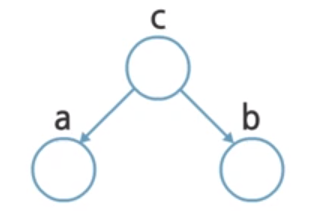In views of $c$, $a$ and $b$ are considered to tail. So it is called Tail-to-Tail dependency.

• Joint Probability: $p(a, b, c) = p(a \vert c) p(b \vert c) p(c)$
• Independence test: $p(a, b) = \sum_c p(a \vert c) p(b \vert c) p(c) \neq p(a) p(b)$
• Notation: $a \not \perp b \vert \emptyset$

In this case, it cannot be decomposed as product of probability of $a$ and probability of $b$ in general. Therefore, $a$ and $b$ are dependent.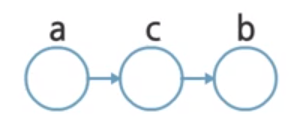It is called Head-to-Tail dependency, since $a$ is considered as head, and $b$ is tail.

• Joint Probability: $p(a, b, c) = p(a) p(c \vert a) p(b \vert c)$
• Independence test: $p(a, b) = p(a) \sum_c p(c \vert a) p(b \vert c) = p(a) p(b \vert a) \neq p(a) p(b)$
• Notation: $a \not \perp b \vert \emptyset$

From the independence test, $a$ and $b$ are dependent, same as before.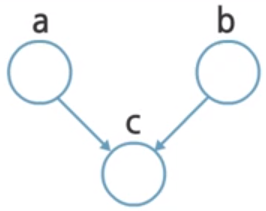Head-to-Head dependency is a little bit different from previous case. Here, $a$ and $b$ are not conditionally dependent on any node.
• Joint Probability: $p(a, b, c) = p(a) p(b) p(c \vert a, b)$